Aarshay Jain — Published On January 19, 2016 and Last Modified On September 4th, 2019

## Introduction

List comprehension is powerful and must know the concept in Python. Yet, this remains one of the most challenging topic for beginners. With this post, I intend help each one of you who is facing this trouble in python. Mastering this concept would help you in two ways:

1. You would start writing shorter and effective codes
2. Hence, your code will execute faster

Do you know List Comprehensions are 35% faster than FOR loop and 45% faster than map function? I discovered these and many other interesting facts in this post.

If you are reading this, I’m sure either you want to learn it from scratch or become better at this concept. Both ways, this post will help you.## 1. What is List Comprehension (LC)?

When I first used LC, it took me down the memory lane and reminded me of the set-builder form. Yes you got me right. Set builder is a concept mostly taught in Class XI in India. (check this). I’ve picked some of easy examples from this text book to explain this concept.

Lets look at some of basic examples:

1. { x^2: x is a natural number less than 10 }
2. { x: x is a whole number less than 20, x is even }
3. { x: x is an alphabet in word ‘MATHEMATICS’, x is a vowel }

Now let’s look at the corresponding Python codes implementing LC (in the same order):

1. `[x**2 for x in range(0,10)]`
2. `[x for x in range(1,20) if x%2==0 ]`
3. `[x for x in 'MATHEMATICS' if x in ['A','E','I','O','U']]`

Looks intuitive right?

Don’t worry even if it feels a bit foggy. Just hang on with me, things will become more clear as we proceed. Let’s take a step back and see what’s happening here. Each of the above example involves 3 things – iteration, conditional filtering and processing. Hence, you might say its just another representation of FOR loop.

In a general sense, a FOR loop works as:

```for (set of values to iterate):
if (conditional filtering):
output_expression()```
```The same gets implemented in a simple LC construct in a single line as:
[ output_expression() for(set of values to iterate) if(conditional filtering) ]```

Consider another example: { x: x is a natural number less than or equal to 100, x is a perfect square }
This can be solved using a for-loop as:

```for i in range(1,101):     #the iterator
if int(i**0.5)==i**0.5: #conditional filtering
print i               #output-expression```

Now, to create the LC code, we need to just plug in the different parts:

`[i for i in range(1,101) if int(i**0.5)==i**0.5]`

I hope it is making more sense now. Once you get a hang of it, LC is a simple but powerful technique and can help you accomplish a variety of tasks with ease. Things to keep in mind:

1. LC will always return a result, whether you use the result or nor.
2. The iteration and conditional expressions can be nested with multiple instances.
3. Even the overall LC can be nested inside another LC.
4. Multiple variables can be iterated and manipulated at same time.

Let’s look at some more examples to understand this in detail.

Predefined functions might exist for carrying out the tasks explained below, but these are good examples to get a grasp of LC.

Note: I will be confining all my codes in functions. It’s generally a good practice as it enhances re-usability but I carry a special motive here which I’ve revealed below.

### Example 1: Flatten a Matrix

Aim: Take a matrix as input and return a list with each row placed on after the other.

Python codes with FOR-loop and LC implementations:

### Example 2: Removing vowels from a sentence

Aim: Take a string as input and return a string with vowels removed.

Python codes with FOR-loop and LC implementations:

```def eg2_for(sentence):
vowels = 'aeiou'
filtered_list = []
for l in sentence:
if l not in vowels:
filtered_list.append(l)
return ''.join(filtered_list)

def eg2_lc(sentence):
vowels = 'aeiou'
return ''.join([ l for l in sentence if l not in vowels])```

Let’s define a matrix and test the results:

```sentence = 'My name is Aarshay Jain!'
print "FOR-loop result: " + eg2_for(sentence)
print "LC result      : " + eg2_lc(sentence)```

Output: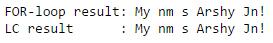### Example 3: Dictionary Comprehension

Aim: Take two list of same length as input and return a dictionary with one as keys and other as values.

Python codes with FOR-loop and LC implementations:

```def eg3_for(keys, values):
dic = {}
for i in range(len(keys)):
dic[keys[i]] = values[i]
return dic

def eg3_lc(keys, values):
return { keys[i] : values[i] for i in range(len(keys)) }```

Let’s define a matrix and test the results:

```country = ['India', 'Pakistan', 'Nepal', 'Bhutan', 'China', 'Bangladesh']
capital = ['New Delhi', 'Islamabad','Kathmandu', 'Thimphu', 'Beijing', 'Dhaka']
print "FOR-loop result: " + str(eg3_for(country, capital))
print "LC result      : " + str(eg3_lc(country, capital))```

Output:I believe with the color convention in place, things are getting pretty much self-explanatory by now. Till now, we have focused on 1 aspect of LC, i.e. its brevity and readability. But that’s not it! LC are also faster in various scenarios as compared to some of the alternatives available. Let’s explore this further.

In this section, we will find out the time taken by LC in comparison to similar techniques. We will also try to unravel the situations in which LC works better and where it should be avoided. Along with runtime, we will compare the readability of the different approaches.

Before jumping into comparing the time taken by different techniques, lets take a refresher on the Python map function.

### map function

It is used to apply a function to each element of a list or any other iterable.
Syntax: map(function, Python iterable)

For example, we can multiply each element of a list of integers with the next number using the following code:

`map(lambda x: x*(x+1), arr)`

### Comparing Runtime

An important part of this exercise is the ability to compare the running time of a code fragment. We will be using %timeit, an in-built magic function of iPython notebook environment. Alternatively, you can use the time or timeit modules.

We will be comparing the runtimes of implementation of same result using LC, FOR-loop and map function. Also, we shall focus on the relative run times and not the absolute values because it is subject to the machine specs. FYI, I am using a Dell XPS 14Z system with following specs:
2nd Gen i5 (2.5GHz) | 4GB RAM | 64-bit OS | Windows 7 Home Premium

### A Simple Example

Lets start with a simple example – squaring each element of a list. The Python codes and runtimes for each of the 3 implementations are:

```#Method 1: For-Loop
def square_for(arr):
result = []
for i in arr:
result.append(i**2)
return result
%timeit square_for(range(1,11))```

Output: 100000 loops, best of 3: 2.69 µs per loop

```#Method 2: Map Function
def square_map(arr):
return map(lambda x: x**2, arr)
%timeit square_map(range(1,11))```

Output: 100000 loops, best of 3: 3.16 µs per loop

```#Method 3: List comprehension:
def square_lc(arr):
return [i**2 for i in arr]
%timeit square_lc(range(1,11))```

Output: 100000 loops, best of 3: 1.67 µs per loop

RunTime: We can clearly see that in this case LC is ~35% faster than for-loop and ~45% faster than map function.
Readability: Both LC and map functions are fairly simple and readable, but for-loop is a bit bulky but not much.

So can we say LC is the fastest method? Not necessary! We can’t generalize our conclusions at this stage as it might be specific to the problem at hand. Let’s consider a slightly tricky example.

### Taking a step forward

Let’s include a catch in above problem. What if we want the square of only even numbers in the list? Now, the three functions would look like:

```#Method 1: For-Loop
def square_even_for(arr):
result = []
for i in arr:
if i%2 == 0:
result.append(i**2)
return result
%timeit square_even_for(range(1,11))```

Output: 100000 loops, best of 3: 2.31 µs per loop

```#Method 2: Map Function
def square_even_map(arr):
return filter(lambda x: x is not None,map(lambda x: x**2 if x%2==0 else None, arr))
%timeit square_even_map(range(1,11))```

Output: 100000 loops, best of 3: 5.25 µs per loop

```#Method 3: List comprehension:
def square_even_lc(arr):
return [i**2 for i in arr if i%2==0]
%timeit square_even_lc(range(1,11))```

Output: 100000 loops, best of 3: 1.74 µs per loop

RunTime: LC is ~25% faster than for-loop and ~65% faster than map function.
Readability: LC is most concise and elegant. Map function became cumbersome with additional lambda function and for-loop is no better.

I think now we have gained some more confidence on LC. Even I can’t wait to compare the runtime of advanced applications. But hang on! I am not totally convinced.

Why is LC faster? After all each technique is doing the same thing on the same scale – iteration, conditional filtering and execution. So what makes LC special? Will it be faster in all scenarios or are these special cases? Let’s try to find out!

### Getting into crux of the matter

Lets compare each algorithm on 3 fronts:

1. Iterating over the list
2. Modifying each element
3. Storing the result

First, lets call a simple function that has no obligation of doing anything and primary role is iteration. The codes and runtime of each technique is:

```#Method 1: For-loop:
def empty_for(arr):
for i in arr:
pass
%timeit empty_for(range(1,11))```

Output: 1000000 loops, best of 3: 714 ns per loop

```#Method 2: Map
def empty_map(arr):
map(lambda x: None,arr)
%timeit empty_map(range(1,11))```

Output: 1000000 loops, best of 3: 2.29 µs per loop

```#Method 3: LC
def empty_lc(arr):
[None for i in arr]
%timeit empty_lc(range(1,11))```

Output: 1000000 loops, best of 3: 1.18 µs per loop

RunTime: FOR-loop is fastest. This is because in a for-loop, we need not return an element and just move onto next iteration using “pass”. In both LC and map, returning an element is necessary. The codes here return None. But still map takes more than twice the time. Intuitively, we can think that map involves a definite function call at each iteration which can be the reason behind the extra time. Let’s explore this at later stage.

Now, lets perform a simple operation of multiplying the number by 2 but we need not store the result:

```#Method 1: For-loop:
def x2_for(arr):
for i in arr:
i*2
%timeit x2_for(range(1,11))```

Output: 1000000 loops, best of 3: 1.07 µs per loop

```#Method 2: Map
def x2_map(arr):
map(lambda x: x*2,arr)
%timeit x2_map(range(1,11))```

Output: 100000 loops, best of 3: 2.61 µs per loop

```#Method 3: LC
def x2_lc(arr):
[i*2 for i in arr]
%timeit x2_lc(range(1,11))```

Output: 1000000 loops, best of 3: 1.44 µs per loop

RunTime: Here we see a similar trend as before. So, till the point of iterating and making slight modifications, for-loop is clear winner. LC is close to for-loop but again map takes around twice as much time. Note that here the difference between time will also depend on the complexity of the function being applied to each element.

An intuition for higher time of map and LC can be that both methods have a compulsion of storing information and we are actually performing all 3 steps for them. So let’s check runtime of for-loop with step 3:

```def store_for(arr):
result=[]
for i in arr:
result.append(i*2)
return result
%timeit store_for(range(1,11))```

Output: 100000 loops, best of 3: 2.55 µs per loop

This is interesting! The runtime of FOR jumps to ~2.5 times just because of storing the information. The reason being that we have to define an empty list and append the result to each in each iteration.

After all 3 steps, LC seems to be the clear winner. But are you 100% sure ? I’m not sure about you, but I am still not convinced. Both LC and map have an elegant implementation (in this case) and I don’t see why map is so slow.

One possible reason could be probably map is slower because it has to make function calls at each step. LC might just be calculating the value of the same expression for all elements. We can quickly check this out. Let’s make a compulsory function call in LC as well:

def x2_lc(arr): def mul(x): return x*2 [mul(i) for i in arr] %timeit x2_lc(range(1,11))

Output: 100000 loops, best of 3: 2.67 µs per loop

Aha! So the intuition was right. When we force LC to make function calls, it ends up taking similar time as the map function.

Let’s summarize our findings now:

1. LC is fast and elegant in cases where simple expressions are involved. But if complex functions are required, map and LC would perform nearly the same
2. FOR-loop is bulkier in general, but it is fastest if storing is not required. So should be preferred in cases where we need to simply iterate and perform operations.

I have compared the runtime of 3 examples we saw earlier and the results are:In the first two cases, LC is faster because they involve storing values in a list. However, in case 3 FOR-loop is faster because it involves dictionary comprehension. Since dictionaries are implemented as hash tables, adding new values is significantly faster as compared to list. Thus we see that the first three examples resonate with our initial findings.

Do you want to explore this further? Why not try the following 3 problems and compare the run-time of FOR-loop implementation vs List Comprehension and we can discuss in comments:

1. Given a number N, find all prime numbers less than N
2. Multiple two matrices
3. Given an integer N, find all possible triangles with unique lengths that can be formed using side lengths <=N

Now, we will look at another facet of LC which will allow you to make it even faster in certain specific applications.

## 3. Generator Expressions (GE)

Never heard of generators in Python? Lets try to understand.

A generator is an iterator but it returns a single value at a time and maintains the iteration count between calls. Its like bowling an over in cricket. The various steps are:

1. Initialization: The captain decides which bowler which bowl and bowler knows he has to deliver 6 balls
2. Ball 1: The bowler bowls for first time, batsman plays the ball, other player field the ball and return to blower.
3. Ball 2-5: Similar procedure is followed
4. Ball 6: This is final delivery and the bowler knows he has to stop after this.

A generator works in similar way. Key things to note about a generator:

1. Like a bowler (in cricket) knows he has to deliver 6 times, a generator knows the #iterations (it can be even be infinite but it would be aware of that)
2. Like a bowler doesn’t throw six balls at the same time, generator also returns values one-by-one on different calls.
3. Like a new bowler is assigned after each over, a generator has to be re-initialized once all iterations are over

Lets consider a simple example – generate integers from 0 to the number N passed as argument to generator.

#Generator definition:

```def my_first_gen(n):
for i in range(n):
yield i```

Now what happens when we print it? Lets try. (Don’t worry about the yield keyword. We’ll come to that in a bit)

`print my_first_gen(10)`

It doesn’t return a list like a traditional for-loop. In fact, it just returns an object. So that way it works is that we have assign this object to a variable and then get numbers using a next statement. In this example, the flow would be:

```gen = my_first_gen(3) #Initialize the generator
print gen.next()```

Output: 0

`print gen.next()`

Output: 1

`print gen.next()`

Output: 2

`print gen.next()`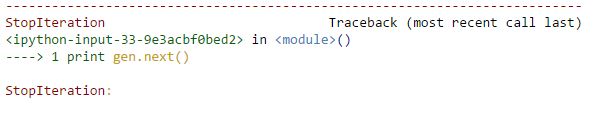I hope its making sense. But how does it know which value to return? That’s where the yield statement comes in. Whatever next is called, generator executes till it encounters a yield statement. The value written after yield will get returned and execution stops. If yield is not found, it returns an error. Let’s consider another example to see the flow of information:

```def flow_of_info_gen(N):
print 'function runs for first time'
for i in range(N):
print 'execution before yielding value %d' % i
yield i
print 'execution after yielding value %d' % i
print 'function runs for last time'```

The outputs for subsequent calls are: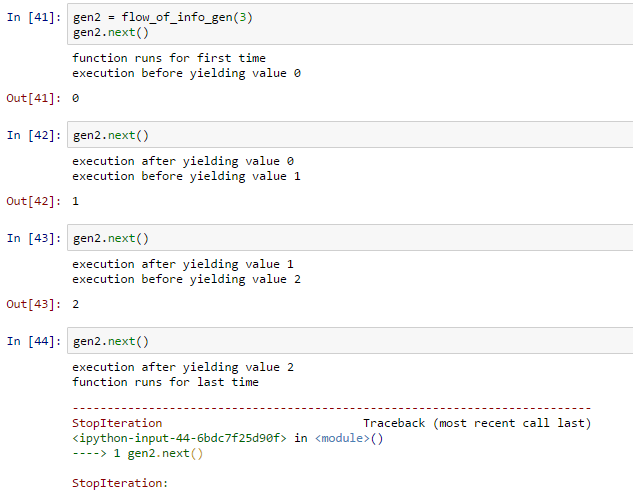I hope things are very much clear now. If you think about an over in cricket, everything will start making sense. There is just one problem, we don’t know when it ends unless it returns an error. One solution is using ‘try and except‘ and check for StopIteration error. But, Python automatically manages this when generators are called within functions which accept an iterator. For example:

```gen3 = my_first_gen(10)
print sum(gen3)```

Output: 45

Note: This starts from current state and works to end. If a next statement is called before sum, it won’t take the numbers already returned into account. Example:

```gen3 = my_first_gen(10)
gen3.next()
gen3.next()
gen3.next()
gen3.next()
sum(gen3)```

Output: 39

Having understood the basics of generators, lets come to Generator Expressions (GE). Essentially, this can simply be done by replacing the [] with (). For example:

```#LC implementation
[x for x in range(10)]```

Output: [0, 1, 2, 3, 4, 5, 6, 7, 8, 9]

```#GE implementation
(x for x in range(10))```

Output: at 0x0000000003F7A5A0>

Again, we can apply some function which takes an iterable as an argument to the LC:

`sum(x for x in range(10))`

Output: 45

Generator is a very useful concept and I encourage you to explore it further.

### Why use Generator in Python?

You must be wondering why should one use a generator! It works one-by-one, has some inherent properties to be kept in mind and it is not compatible with many functions and even additional libraries.

The biggest advantage is that generators have very low memory requirements as they return numbers on the fly. On the other hand, using simple LC will first create a list in memory and then sum it. However, generators might not perform well on small problems because they make many function calls making it slower. It should be used for solving computations which are too heavy on memory.

Consider following functions:

```def sum_list(N):
return sum([x for x in range(N)])
def sum_gen(N):
return sum((x for x in range(N)))```

I ran these functions for 3 different values of N and the runtimes are illustrated below:Conclusions:

1. For small N, GE are relatively slower than LC. This is because of high multiple calls are required in GE but LC doesn’t require much space in memory.
2. For moderate N, GE perform almost the same as LC. GE requires high number of calls and LC needs large memory block.
3. For very high N, generators are drastically powerful as LC takes ~70 times of the time taken by GE. This is because LC uses up a very large chunk of RAM and processing becomes very slow.

## 4. Using this concept in Data Science

Moving towards the end, lets discuss some applications of these concepts immensely useful for a data scientist. A quick recap, remember the general structure of LC:

` [ output_expression() for(set of values to iterate) if(conditional filtering) ]`

### Example 4: Reading a list of list

You might have faced situations in which each cell of a column in a data set contains a list. I have tried to simulate this situation using a simple example based on this data. This contains a dataframe having 2 columns:

1. personID: a unique identifier for a person
2. skills: the different games a person can play
```Lets load the dataset:
import pandas as pd
print data```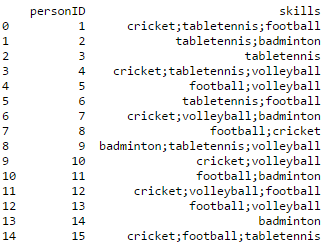Here, you can see the different sports a person plays mentioned in the same column. To make this data usable in a predictive model, its generally a good idea to create a new column for each sport and mark it as 1 or 0 depending on whether the person plays it or not.

First step is to convert the text into a list so that individual entries can be accessed.

```#Split text with the separator ';'
data['skills_list'] = data['skills'].apply(lambda x: x.split(';'))
print data['skills_list']```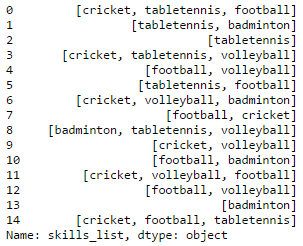Next, we need a unique list of games to identify the different number of columns required. This can be achieved through set comprehension. Sets are collection of unique elements. Refer to this article for basics of python data structures.

```#Initialize the set
skills_unq = set()
#Update each entry into set. Since it takes only unique value, duplicates will be ignored automatically.
skills_unq.update( (sport for l in data['skills_list'] for sport in l) )
print skills_unq```Note that, here we have used generator expression so that each value gets updated on the fly and storing is not required. Now we will generate a matrix using LC containing 5 columns with 0-1 tags corresponding to each sport.

```#Convert set to list:
skills_unq = list(skills_unq)
sport_matrix = [ [1 if skill in row else 0 for skill in skills_unq] for row in data['skills_list']  ]
print sport_matrix```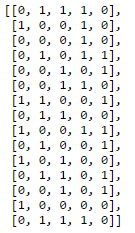Note that, here again one instance of LC has been nested into another. The inner LC is highlighted using bold square brackets. Please follow the color convention for understanding. If still unclear, I recommend trying a for-loop implementation and then converting to LC. Feel free to leave a comment below in case you face challenges at this stage.

The last step is to convert this into Pandas dataframe’s column which can be achieved as:

```data = pd.concat([data, pd.DataFrame(sport_matrix,columns=skills_unq)],axis=1)
print data```### Example 5: Creating powers of a columns for Polynomial regression

Polynomial regression algorithm requires multiple powers of the same variable, which can be created using LC. As much as 15-20 powers of the same variable might be used and modeled along with Ridge regression to reduce overfitting.

Let’s create a 1-column data set for simplicity:

```data2 = pd.DataFrame([1,2,3,4,5], columns=['number'])
print data2```Lets define a variable “deg” containing the number of degrees required. Keeping it dynamic is generally a good practice to allow flexibility. Our first step is to create a matrix containing the different powers of ‘number’ variable.

```#Define the degree:
deg=6
#Create the matrix:
power_matrix = [ [i**p for p in range(2,deg+1) ] for i in data2['number'] ]
print power_matrix```Now we will add this to the dataframe similar to the previous example. Note that, we need a list of column names in this case and again LC can be used to get it fairly easily:

```cols = ['power_%d'%i for i in range(2,deg+1)]
data2 = pd.concat([data2, pd.DataFrame(power_matrix,columns=cols)],axis=1)
print data2```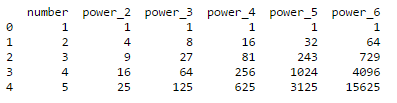Thus the powers have been successfully added.

### Example 6: Filtering column names

Personally, I have faced this issue numerous times of selecting a subset of columns in a dataframe for the purpose of setting as predictors in a predictive model. Let’s consider a situation where the total columns are:

`cols = ['a','b','c','d','a_transform','b_transform','c_transform','d_power2','d_power3','d_power4','d_power5','temp1','temp2']`

These can be understood as:

1. a,b,c,d: originals columns as in raw data
2. a_transform,b_transform,c_transform: features made after applying certain transformations like taking log, square root, combining categories, etc.
3. d_power2,d_power3,d_power4,d_power5: different powers of the same variable for polynomial or ridge regression
4. temp1, temp2: intermediate variables created for performing certain calculations

Depending on the analysis being performed or the model being used, there might be different use cases:

1. Select only transformed variables
2. Select different powers of same variable
3. Select combination of above 2
4. Select all except temporary variables

These can be done using following code.

```col_set1 = [x for x in cols if x.endswith('transform')]
col_set2 = [x for x in cols if 'power' in x]
col_set3 = [x for x in cols if (x.endswith('transform')) | ('power' in x)]
col_set4 = [x for x in cols if x not in ['temp1','temp2']]
print 'Set1: ', col_set1
print 'Set2: ', col_set2
print 'Set3: ', col_set3
print 'Set4: ', col_set4```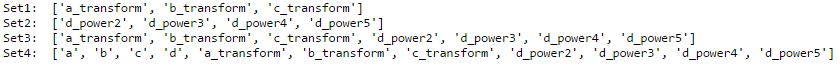## End Notes

This brings me to an end of this tutorial.  Here we took a deep dive into List Comprehension in Python. We learned about time advantage of LC, comparison of LC with map and FOR loop, generator expressions and explored some advanced applications.

If you have reached this point, I believe that now you would be able to appreciate the importance of LC. You should try to incorporate this technique in daily practice. Though it might be a bit time consuming in beginning, but trust me you are going to enjoy it as you proceed further.

Did you find this article helpful ? Did I miss something ? Do you have some more interesting applications where you think LC will be useful? Please share your comments and I will be more than happy to discuss.

### If you like what you just read & want to continue your analytics learning, subscribe to our emails, follow us on twitter or like our facebook page.###### Aarshay Jain

Aarshay graduated from MS in Data Science at Columbia University in 2017 and is currently an ML Engineer at Spotify New York. He works at an intersection or applied research and engineering while designing ML solutions to move product metrics in the required direction. He specializes in designing ML system architecture, developing offline models and deploying them in production for both batch and real time prediction use cases.

## 10 thoughts on "Tutorial – Python List Comprehension With Examples"###### Jason Hollis says:January 20, 2016 at 1:28 am
Here are a couple of typos i found in the code examples: 1. skills_unq.update( (sport for l in data['skills_list') for sport in l) ) is missing ']' after 'skills_list' 2. data = pd.concat([data, pd.DataFrame(t,columns=skills_unq)],axis=1) needs the 't' replaced with 'sport_matrix' Reply###### Aarshay Jain says:January 20, 2016 at 4:07 am
Hello Jason, Thanks for pointing this out. I have made the required changes. I'm glad you took out the time to run the codes. Thanks again! Hope you found the article useful. Cheers, Aarshay Reply###### Jeff Weakley says:January 20, 2016 at 8:01 pm
Analytics Vidhya is really one of the most useful, practical newsletters/blogs I get. I really appreciate this article, and the ongoing string of great info you guys put out. Much thanks. Reply###### Aarshay Jain says:January 21, 2016 at 4:04 am
Thanks Jeff! We are trying our best to keep up the good work. :) Reply###### Meethu Mathew says:January 21, 2016 at 6:32 am
This blog is very helpful and very easy to understand. skills_unq.update( (sport for l in data['skills_list']) for sport in l) ) Could you plz explain this bit more ? Reply###### Aarshay Jain says:January 21, 2016 at 10:10 am
Hi Meethu, Thanks for reaching out. Let me rephrase the problem first. The aim is to find the unique skills from a column in which 'each entry is a list of skills' Actually the code should be- skills_unq.update( (sport for l in data[‘skills_list’] for sport in l) ) Different parts of code: 'update': function used to add the values of a set into another. Note that since sets can contain only unique values, the duplicates will be automatically rejected. 'for l in data['skills_list']' - this will iterate through each cell in the column 'skills_list' of the data frame 'data'. Note that this is not enough because it would return a list. 'for sport in l': this extracts each element of the list All this is returned one by one to the update command so that no storage is required. Hope this makes sense. Cheers, Aarshay Reply###### Nandan Padar Chandrashekar says:March 20, 2016 at 5:36 am